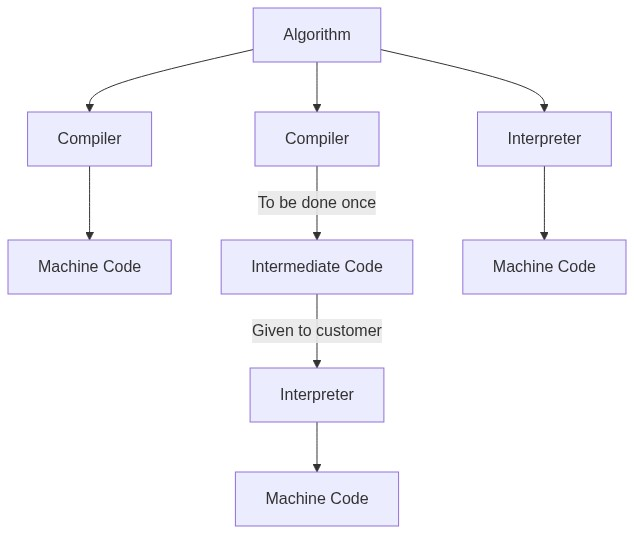# Computing n!

Imperative style:

``````for i in range(1, n+1):
factorial=factorial * i
``````

Functional Style

``````def factorial(n):
if n==1:
return 1
else:
return n*factorial(n-1)
``````

# What is a functional language

A style of programming where the building block of computation is an application to arguments.

# Side effects

Side effect - Modify some (internal/hidden) state as well as returning a value

An example of this:

`y1=f(1)`

`y2=f(2)`

You would expect `y1==y2`, however if f has an internal state that affects the answer this will not be true

``````state=0
def f(n):
global state
state +=1
return n+ state

print(f(1))=> "2"
print(f(1))=> "3"
``````

# A functional approach

• Forbid variable assignment and side effects in the language “pure functional”

• Makes reasoning about code simpler (for humans and compilers)

• A new programming paradigm: takes some time to get used to

# Why programming languages

## Abstracting from the machine

\$b=a+3\$

``````mov addr_a, reg1; Load address of a into reg1
add 3, reg1, reg2; add 3 to reg1 and write into reg2
``````
• Explicit about what is going on

• Obfuscates algorithm from implementation

• Not portable (would need different instructions for different hardware e.g. different registers)

• Not easy to modify

• Not succinct

## Programming languages

• Allow writing code to an abstract machine model

• A translator of some kind (perhaps a compiler) transforms this code into something that executes on some hardware (sometimes this hardware is a virtual machine)

• Some virtual machines are “hybrid”: they do just-in-time compilation

• The Haskell distribution we will use has both Compiler and Interpreter mode• Micro-architecture just reads an instruction stream

• Note easy to program complex algorithms in such a “language” so use abstractions leading to high level languages

• Features driven by programming paradigm considerations, domain knowledge, wanting to target particular hardware…

• Compiler or interpreter maps this language onto machine instructions

• Therefore we need a formal specification

# Example

``````filter:: (a -> Bool) -> [a] -> [a]
filter p [] = []
filter p (x:xs)
| p x   = x: filter p xs
| otherwise = filter p xs
``````
• Higher order

• Polymorphic (works for all types a) (functions that take functions as parameters)

• Function defined with recursion and pattern matching

# Syntax and Semantics

Syntax - What are valid sentences (expressions) in a language?

Semantics - What do these valid sentences (expressions) mean?

# Naming Requirements

• Can have characters in function names, for example `x’`

• s at the end to show a list

• lowercase letter to start

``````-- Comment like this